GOLD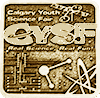#### COVID-19 mathematical model simulation of WDP's infectious level

This mathematical model is called S.I.R (Susceptible, Infectious, Recovered). What I am doing is taking this model and putting it into a computer simulation for it to run for any given days to see how much people will be in each category!

### Problem

COVID-19 is an international problem! This problem is widespread around the world, it has been ongoing for around 1 year now with almost 2 million deaths . As many scientists and political structures do, they research, "control", and try to develop a vaccine! The vaccine and chemicals I will not dive deep into but all I can say is that it has been going well lately with vaccine development! Now, back to the problem at hand. Though, in the midst of the virus when no vaccination was yet available, getting information on the acts of transmission is very wise, to say the least. With this project, I have made a computer program that simulates the coronavirus transmission between the facility members and the students in W.D.P school. In this program, we are simulating through the population the numbers of S.I.R in a reality-based atmosphere. We will see what the results will be if say we made the facility members work on hygiene, protection, and social distancing! It's been hard keeping track of the virus as usual, it will never be 100% accurate but this is a good estimate. This is the whole problem, so naturally I wanted a satisfying answer! With the supervision of my mother and estimation with code writing, I have made a reasonable estimate!

### Method

Mathematics of S.I.R

The S.I.R model is the simplest of the simplest when simulating diseases! While operating the model, you must split the population into 3 main groups, S (Susceptible). I (Infected), R (Recovered). This model grants you the ability to calculate how many people are in each section by time through specific equations!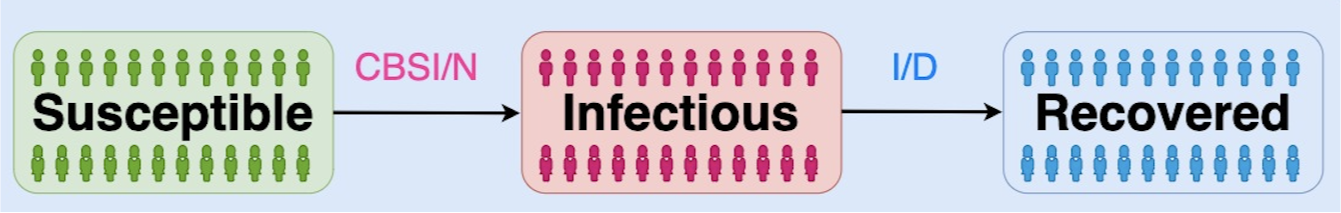Imagine that S.I.R stands for the total of the people that are either in Susceptible, Infected, and/or Recovered. Those 3 together makes a total population of N, the initial total of S.I.R on day 1 is:

S1: 999

I1: 1

R1:0

Equations for New Infected day 1: S1*I1*C*B/N

Equation for New Recovery day 1: I1/D

Using this, it is now possible to solve the numbers for day 2

Day 2:

S2=S1-New Infected

I2=I1+New Infected-New Recovered

R2=R1+New Recovered

New Infected day 2: C*B*S2*I2/N

New Recovered day 2: I2/D

This will go on and on for however long you would like! You will have to repeat the process again and again.

Explanation:

Factors

S1 came from the whole population of the given facility. (in this case a school) (Numbers or N is 1000) So the requirements needed must be met (requirement is at least 1 person infected and is not socially distanced) then the school outbreak will start with day 1. There will be very few people that are infected on the first day!

Equation

Now if we take the total number of people that are susceptible (or S) which is 999, then we'll just assume that there will be 5 more infected (I) each day by increasing! Though we’ll just assume that each day 1 infected converts to recovered (R). So on the first day, it is reasonable to assume that there is 999 S, 1 I, and 0 R.

It can be also written down as.

(S)1000-1=999   (I)0+1    (R) 0

Day 2

Explaining

Factors

As said in the first day I will just assume that the I’s will gain 5 each day but lose 1, while R is gaining 1 each day from I’s losses, S will have whatever is remaining of the 5 that I took.

Equation

So if we take S’s number on the previous day which is 999 and subtract it by 5 which is I’s assumed gainings in 1 day! I on the first site will seem to have 6 but if we take the subtraction of 1 into consideration for R then there will be 5 for I. S on the other hand has 994 now because of subtracting the 5 for I. R will now have 1 cause of the first child from day 1 recovering and is now in the R section!

This equation will look like.

S2 999-5=994   I2 1+5-1=5   R2 0+1=1

In total this whole thing will go on as long as you please in this order as of now!

• Dictionary

Introduction to Contacts per day and transmission possibility

C=Contacts per day

Now that we’ve gone through the assumptions,  we’ll have to calculate the actual amount of people! I have calculated from the student's perspective they will be contacting around 11 human beings a day! Then that will make 11 contacts per day!

B=Transmission probability

It isn’t exactly obvious with this one, you have to do a lot of background research and work for you to find out just the general. For that, I’ll just leave it out because of the endless possibilities!

D=Default Recovery Days

(The usual amount of time for a person to recover)

So, in total, you calculate everything like this.

S x I x C x B/(S+I+R)=?

Newly recovered=I/d

Example: S (999) * I (1) = 999

999 * C (11) = 10,989

10,989 * B (0.1, or divide by 10) 0.1 or /10 = 1,098.9

1,098.9 divide N or (S+I+R) = 1.0989

And that is the mathematical way of calculating and converting everything by S.I.R!

(Please refer to the logbook where I have manually calculate the first 5 days of the WDP population)

### Research

-Computer Program

We could manually calculate everything if we wanted to but it takes too much time, not to mention very easy to make a mistake. So in response to that, I have made a computer program for this! You can calculate each and every day, this program is in Scratch.

• Computer program

Although we can manually calculate every day's population in the category of susceptible, infectious, and recovered, it is too tedious. So, I have built a computer program to calculate the values of S, I, and R every day by Scratch.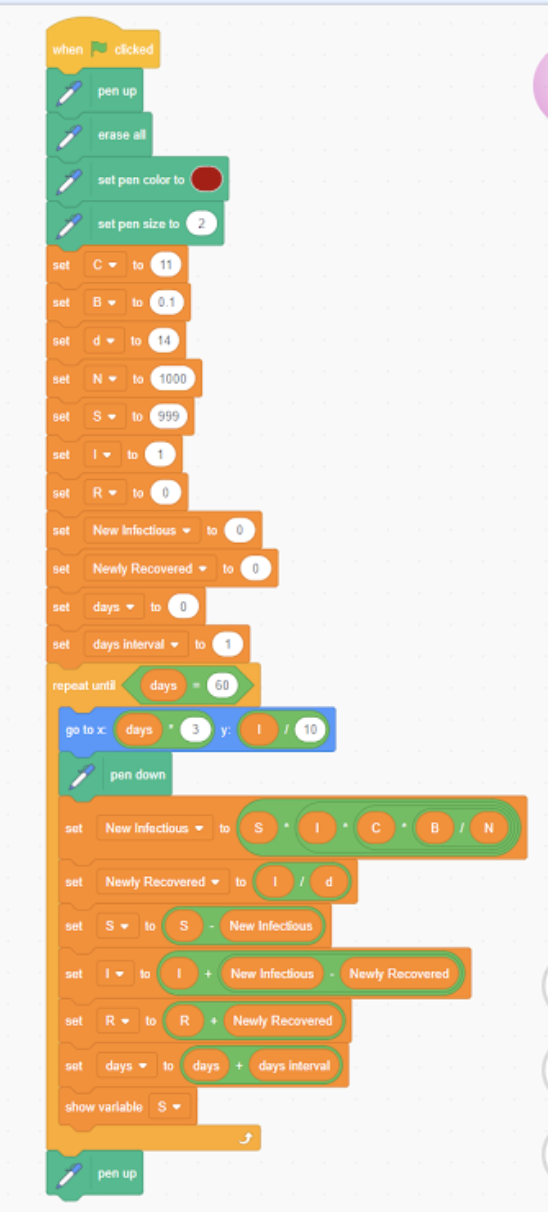-Scenarios/Scenes

I will be explaining and summarizing the Coronavirus effects of 5 separate levels, it contains: Careless (C=11), Action level 1 (C=8), Action level 2 (C=6), Action level 3 (C=4), Action level 4 (C=2).

Careless: Careless: If the school does absolutely nothing even after it's been 100% confirmed that the child or staff has Coronavirus and they don't do anything about it, that would be careless. If that were to happen with C (Contact rate) at 11, then the school will skyrocket immediately no doubts.

Intervention level 1: So the staff finally realized the mistake? Good, now the C is (Potentially) at 8! Nearly two-thirds of what it used to be. Social distancing finally started to show its effects! Believe me when I say that this is only the beginning but this beginning is finally starting to see light!

Action level 2: The school facility members continue to be diligent and understanding while helping fight the virus! (C=6)

Action level 3: The school facility members continue to be diligent and understanding while helping fight the virus! (C=4)

Action level 4: The school facility members continue to be diligent and understanding while helping fight the virus! (C=2)

### Data

C （Contact Rate)=11 persons per day

Reason: There is absolutely no scientific evidence about the contact rate being 11 but as a member/student of the school, I believe I have the right to say why I think the contact rate is around 11. The first 8 comes from my table and neighboring tables cause the usual number of people at the tables is 4-5, so if that adds up my table and the neighboring table adds up to 8. The next 2 are my closest and only friends, I hang out with them at all breaks so it should be counted in. The last one is of course the teacher. I believe that the minimum is 8 cause if the lowest table numbers of their table and the neighboring table be 4 then that will make 7 on its own. The last one is the teacher again. So 8 will be the minimum, the maximum is harder to predict because the student's reputation and status will be taking charge of how many people they contact daily.

B (Transmission probability)=0.1 

Reason: This, unlike the other one has scientific proof of it being 0.1. It seems that after 10 times contact of said given person, you will have a 1 rate chance of getting infected. Well only if said given person is infected that is!

N (Total population)=1000

Reason: It has been confirmed before that there are around 900 students in the school. The other 100 is a VERY rough estimate of the staff in the facility!

D (Days)=14 days 

Reason: 14 days is the default for how long it takes to recover, though it could take longer or shorter depending on how that person's immune systems work.

### Conclusion

This project genuinely helped me understand viruses and how they spread! The conclusion will be completely based on the "Research", these 5 scenarios were explained in Research, it had been calculating to 60 days with the starter as the first person.

This project has helped me understand how viruses spread! The whole conclusion will be based on the Research tab. Finally, those 5 scenarios defined in the section of research have been calculated forward 60 days starting from the first infected person occurred in the WDP school.

Careless: In short, carelessness will lead to downfall, the schools I will skyrocket by a landslide, the situation is careless. The usual amount of Contact is 11, which can definitely cause an outbreak.

The population of S with the scene of C=11, each y unit stands for 10 people, one unit f the x-axis represents 8 hours total.The population of S with the scene of C=11, each y unit stands for 10 people, one unit f the x-axis represents 8 hours total.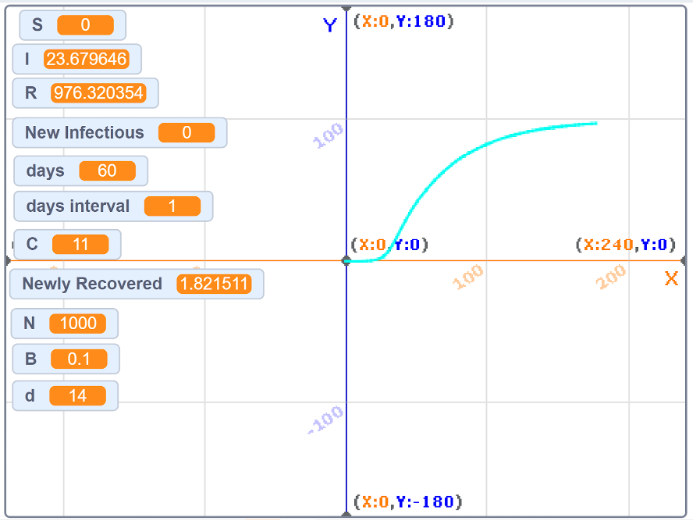The population of S with the scene of C=11, each y unit stands for 10 people, one unit f the x-axis represents 8 hours total.

• Action level 1 (C=8)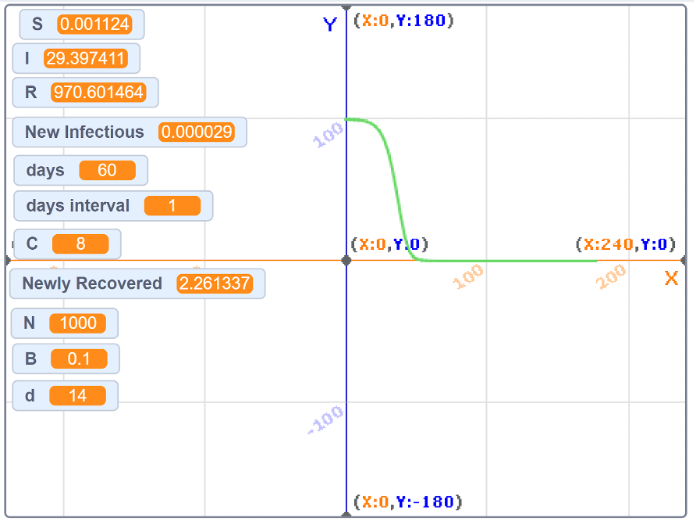The population of S with the scene of C=11, each y unit stands for 10 people, one unit f the x-axis represents 8 hours total.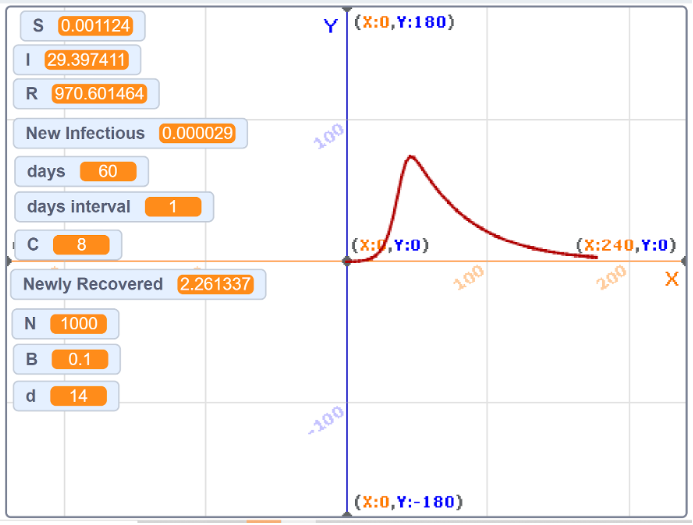The population of S with the scene of C=11, each y unit stands for 10 people, one unit f the x-axis represents 8 hours total.The population of S with the scene of C=11, each y unit stands for 10 people, one unit f the x-axis represents 8 hours total.

• Action level 2 (C=6)The population of S with the scene of C=11, each y unit stands for 10 people, one unit f the x-axis represents 8 hours total.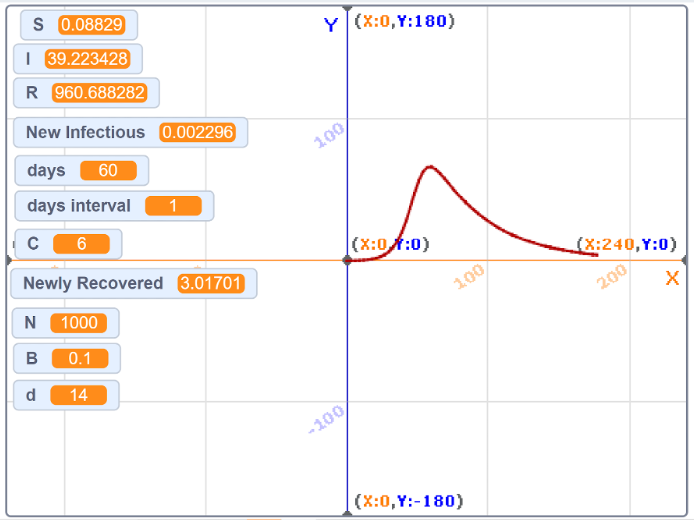The population of S with the scene of C=11, each y unit stands for 10 people, one unit f the x-axis represents 8 hours total.The population of S with the scene of C=11, each y unit stands for 10 people, one unit f the x-axis represents 8 hours total.

• Action level 3 (C=4)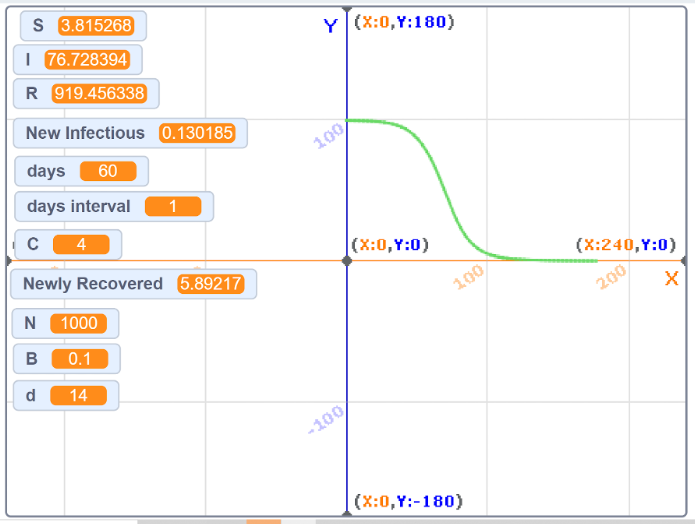The population of S with the scene of C=11, each y unit stands for 10 people, one unit f the x-axis represents 8 hours total.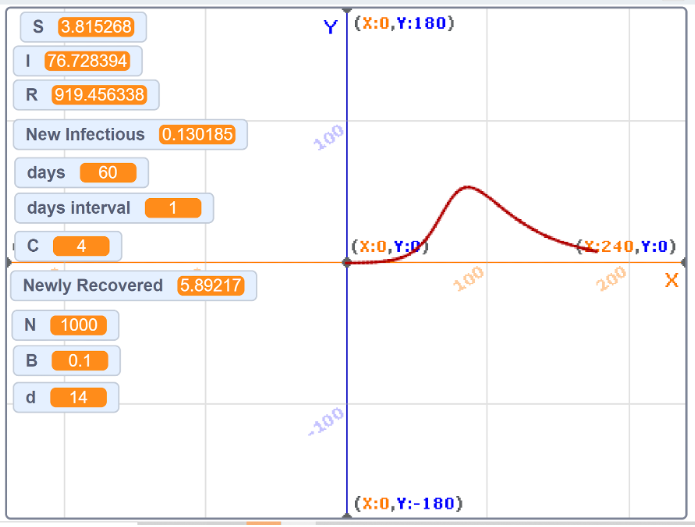The population of S with the scene of C=11, each y unit stands for 10 people, one unit f the x-axis represents 8 hours total.The population of S with the scene of C=11, each y unit stands for 10 people, one unit f the x-axis represents 8 hours total.

• Action level 4 (C=2)The population of S with the scene of C=11, each y unit stands for 10 people, one unit f the x-axis represents 8 hours total.The population of S with the scene of C=11, each y unit stands for 10 people, one unit f the x-axis represents 8 hours total.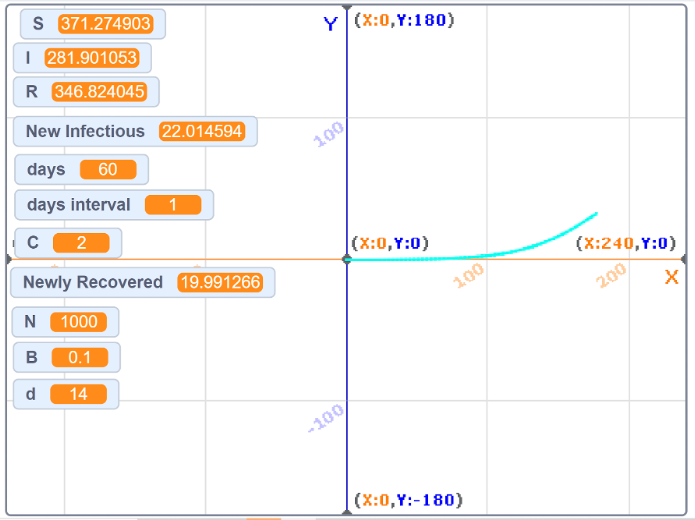-Analysis Results

All the results we've seen so far were from the computer program as you can see from the screenshots above, in the results, we see that in order to control the virus we need to apply less C (Contact rates). There is definitely an outbreak when the C was 2, we can see clearly that this outbreak is definitely less serious than all the other ones. In conclusion, using logic and decreasing the contacts will help prevent a big outbreak, it also can be used as a transmission strategy!

-Future Work

Due to the time, this time I couldn't fulfill something else, the vaccination route, maybe we could do this again and add vaccination next time!

### Acknowledgement

I thank my supervisor, parent, and expert scientist (Xiaoyan Li/Mother) for their help in my project, I also hope that this project can flourish the way we planned it to be!